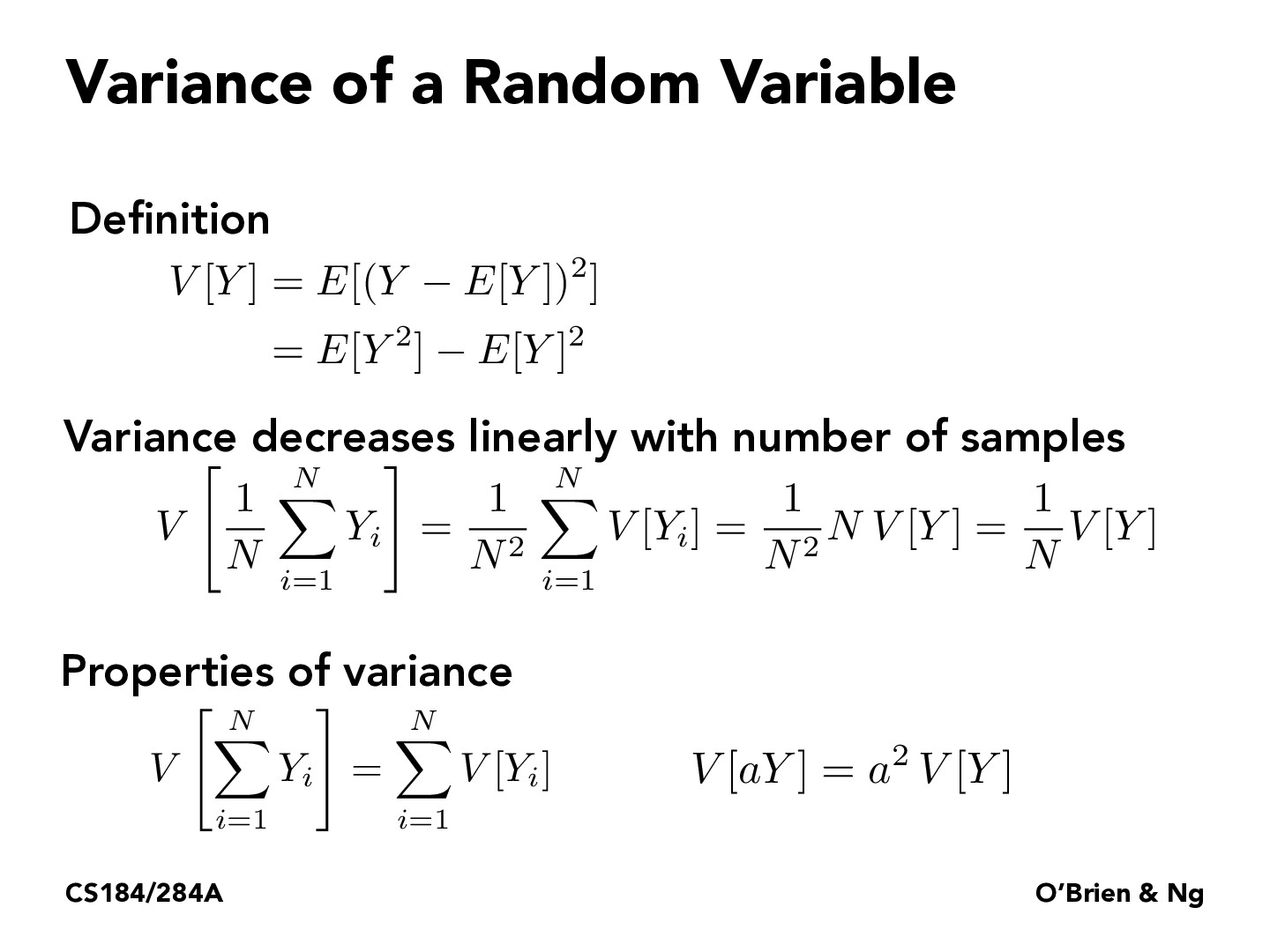Lecture 12: Monte Carlo Integration (27)jacklishufan

These slides demonstrate that the variance decreases linearly with samples, so the error is O(1/sqrt(N)). However, I find this a bit unconvincing because in numerical integration, the errors are not estimated in terms of probability but the local Taylor expansion of the desired function that offers a strong guarantee on the rate of convergence. However, here the "error" is defined on V[Y]. where Y=f(x)/p(x). Since f is deterministic, this implies that all we are measuring depends on our choice of distribution p(x), which can be arbitrary. Is there an equivalent between these 2 types of error measurements? I believe this is also elaborated in the sample-distribution fit in follow-up slides, where the error can be very big or small depending on the choices of p(x).Sicheng-Pan

The properties of variance described in this slide is invariant with respect to the underlying random variable. For a given $p(x)$, we have corresponding $Y_1,...,Y_n$, and the variance for the average of these random variables scales with the inverse of the sample count.jacklishufan

Now suppose $x~N(0,1)$ and $F(x) = C f(x)$ where $C$ is constant and $f$ is the normal p.d.f.. Then $Y=Cf(x)/f(x)$ has mean $C$ and variance 0. However, if we take $x~N(100,100)$ then clearly $Y=Cf(x)/f((x-100)/100)$ is not determinstic and variance is not zero. These two setups seem to have drastically different error behavior. It is true that in both case the error is proportional to the inverse of $\sqrt{N}$ , however they also dependent on some choice of $p(x)$, which is an "additional source" of error that does not exist in typical numerical integralstexus

A lot of these variance properties are actually quite intuitive except for the linearity!

You must be enrolled in the course to comment